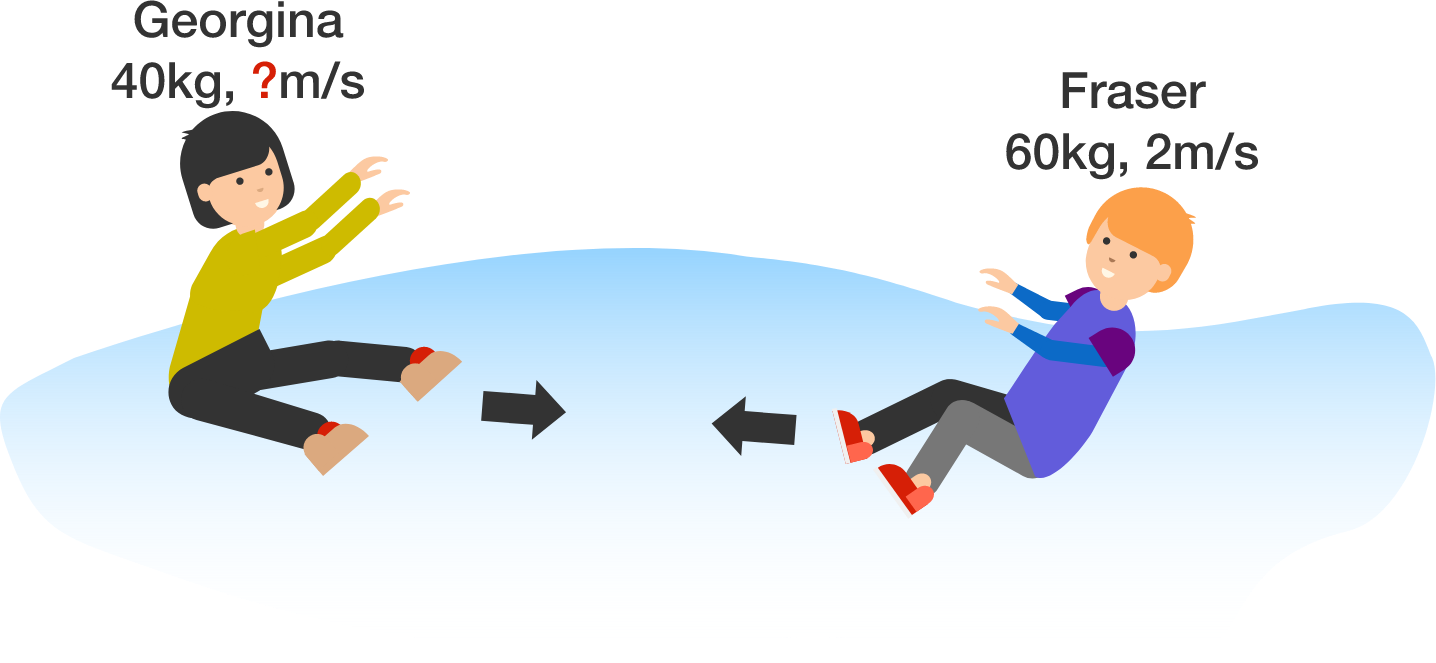# Slipping and Sliding (Snowy Shenanigans III)

Fraser and Georgina are brother and sister and are playing outside in the snow. They come across a sheet of ice and want to slide on it to collide with each other, at which point they will cling together and let themselves go in whichever direction they go. Fraser's mass is $60\text{ kg}$ and Georgina's mass is $40\text{ kg}.$ Fraser travels at $2\text{ m/s},$ whereas Georgina travels at $v\text{ m/s}.$

Fraser: "For us to not move after the collision, it must be true that $v = \frac{2}{3}.$"
Georgina: "If $v > 3$, I will move backwards after the collision."

Just then, their neighbors Owen and Paddy appear to weigh in on the debate.

Owen: "It doesn't matter what $v$ is. Fraser will move forward as he is heavier."
Paddy: "Fraser will move forwards if $v < 3.$"

Who is correct?

Details and Assumptions:

• The ice can be assumed to be smooth (i.e. frictionless).
• The collisions can be assumed to conserve momentum.This problem is part of the Snowy Shenanigans set.

×